# Test Prep Plan - Take a practice test

Take this practice test to check your existing knowledge of the course material. We'll review your answers and create a Test Prep Plan for you based on your results.
How Test Prep Plans work
1
2Based on your results, we'll create a customized Test Prep Plan just for you!
3Study smarter
Study more effectively: skip concepts you already know and focus on what you still need to learn.

# Limits & Continuity in Differential Calculus Chapter Exam

Exam Instructions:

Choose your answers to the questions and click 'Next' to see the next set of questions. You can skip questions if you would like and come back to them later with the yellow "Go To First Skipped Question" button. When you have completed the practice exam, a green submit button will appear. Click it to see your results. Good luck!

### Page 1

#### Question 1 1. Using the squeeze theorem, what is z?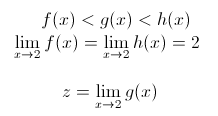#### Question 2 2. Using the squeeze theorem, what is z?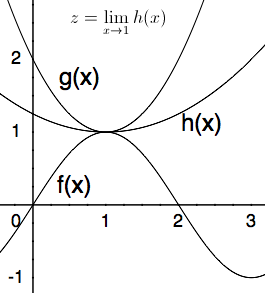#### Question 3 3. What is the limit of the following: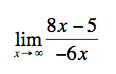#### Question 4 4. Of the three limits shown below, which can be evaluated using L'Hopital's Rule?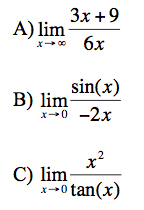### Page 2

#### Question 9 9. Evaluate the limit below.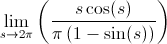#### Question 10 10. Evaluate the limit below.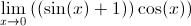### Page 3

#### Question 11 11. Evaluate the limit shown below: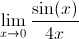#### Question 12 12. Evaluate the limit shown below: (Hint: You can use L'Hopitals's Rule more than once!)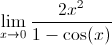#### Question 13 13. Use the graph to solve for z.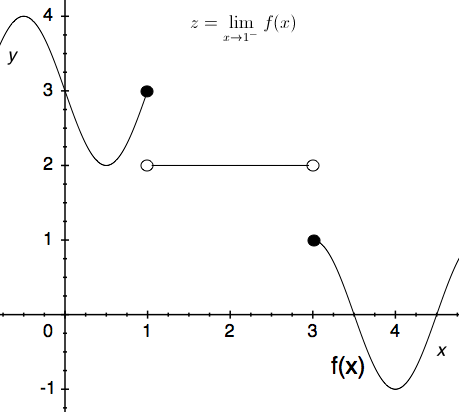#### Question 14 14. Use the graph to solve for z.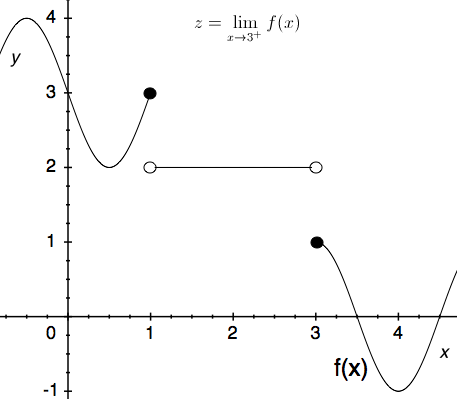#### Question 15 15. What are the regions of continuity for y defined as?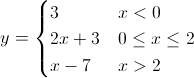### Page 4

#### Question 16 16. What are the regions of continuity in this graph of f(x)?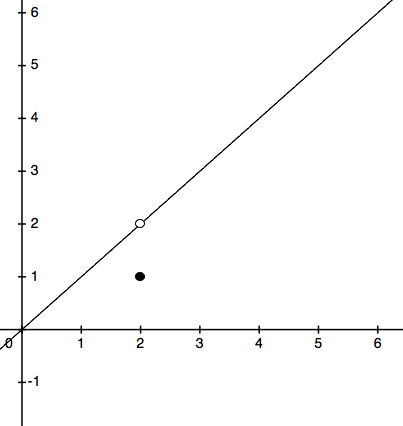#### Question 17 17. Use the graph to solve for z.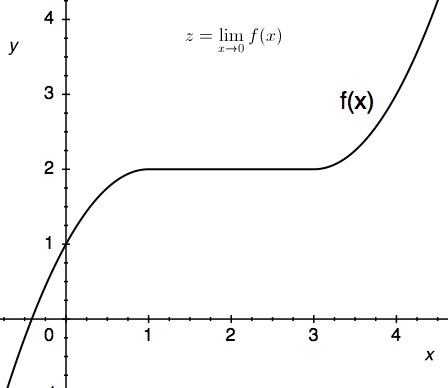#### Question 18 18. Using the graph, find the value of z, then add 1.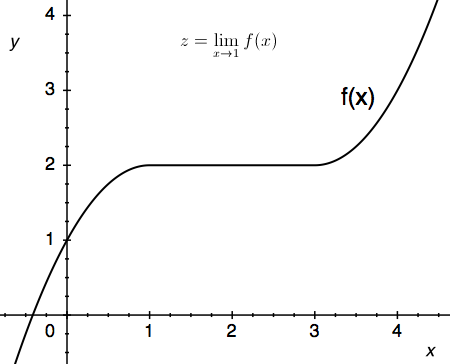#### Question 19 19. The floor function is graphically represented as a stepwise function. What is the limit of f(x) = floor(x) as x approaches 4?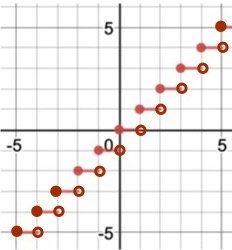### Page 5

#### Question 21 21. Using the given data, predict the likely value of the continuous function z.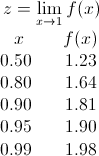#### Question 22 22. Use the given graph and formula to solve for z.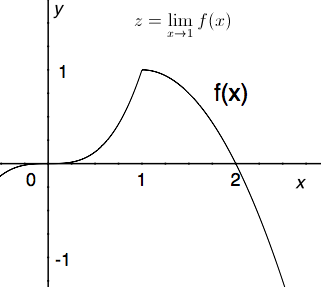#### Question 23 23. Given the values of the continuous function f(x) below, how many solutions will there be to f(x)=4.1?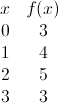#### Question 24 24. Consider the function f(x) below. Considering the intermediate value theorem and the vertical asymptote at x=0, is the following statement true or false? For any number C greater than 0, there is a positive value of x that satisfies the equation f(x)=C.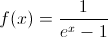### Page 6

#### Question 27 27. Using the squeeze theorem, what is z?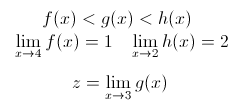#### Question 28 28. According to L'Hopital's rule, which of the following statements MUST be true for the equation shown below to hold? A) f(x) and g(x) approach 0 or infinity B) f'(C) does not equal 0 C) g'(x) does not equal 0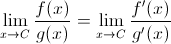#### Limits & Continuity in Differential Calculus Chapter Exam Instructions

Choose your answers to the questions and click 'Next' to see the next set of questions. You can skip questions if you would like and come back to them later with the yellow "Go To First Skipped Question" button. When you have completed the practice exam, a green submit button will appear. Click it to see your results. Good luck!

Support